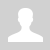Python. Logic operations AND, OR, NOT

11.1.1.8 use logical operations AND, OR, NOT in selection structure

Python. Logic operations AND, OR, NOT

Boolean logic. Truth tables.

 Operator Description Example and Returns True if both statements are true x >= 5 and  x < 10 or Returns True if one of the statements is true x < 5 or x > 15 not Reverse the result, returns False if the result is true not(x >= 5 and x < 10)

Priority of logic operations:

High to low:
1. not
2. and
3. or

Question. Define the sequence of logic operators:
A and B or not C
1. Q1 = not C
2. Q2 = A and B
3. Q = Q1 or Q2

Example 1

```username = input("Username: ") password = input("Password: ") if username == "root" and password == "123456":     print("User logged in.") else:     print("Incorrect username or password.")```

Example 2

```email = input("Username: ") phone = phone("Phone: ") if email == "ex@gmail.com" or phone == "+77771234567":     password = input("Password:")     if password == "123456":         print("User logged in.")     else:         print("Incorrect password.") else:         print("Incorrect email or phone.")```

Example 3

```email = input("Username:") phone = phone("Phone") if not(email == "ex@gmail.com" or phone == "+77771234567"):     print("Incorrect email or phone number.") else:     password = input("Password:")     if password == "123456":         print("User logged in.")     else:         print("Incorrect password.")```

Questions:

Exercises:

Ex. 1. Define the sequence of actions in logic expression.

Ex. 2. Give result of logic operations (True/False)

Категория: Programming languages | Добавил: bzfar77 (19.09.2021)
Просмотров: 272 | Теги: Boolean, Or, Logic, False, and, Condition, true, not, Python | Рейтинг: 5.0/1
 Всего комментариев: 0